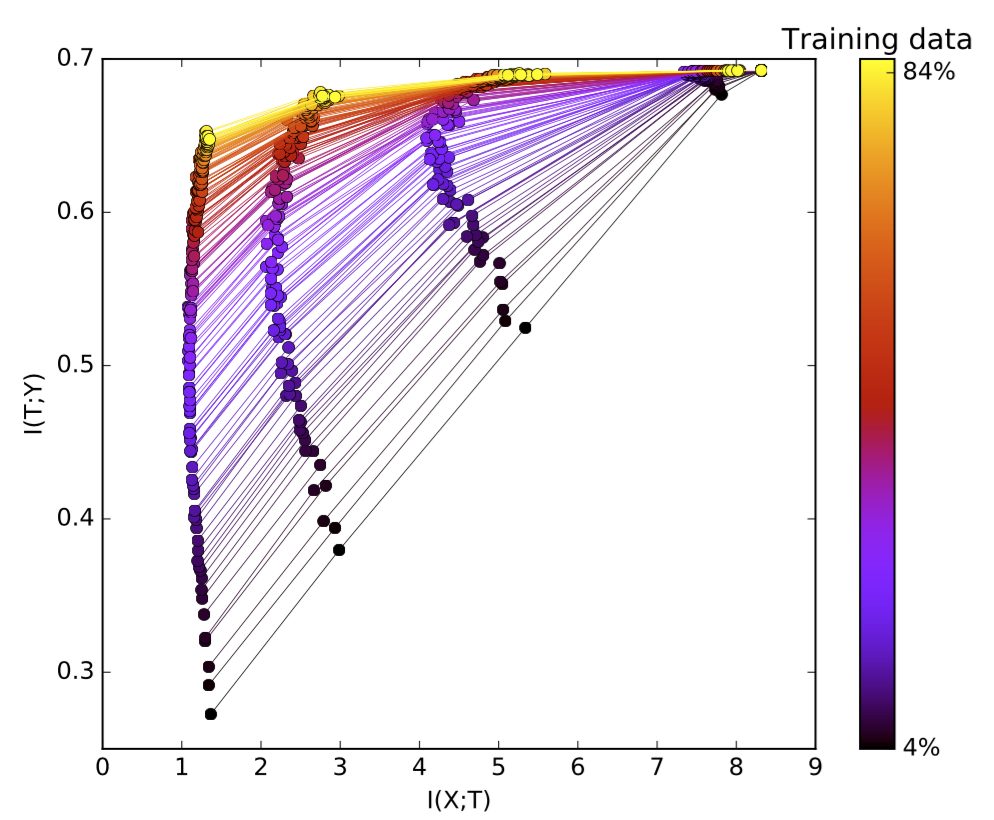## 用信息论剖析深度学习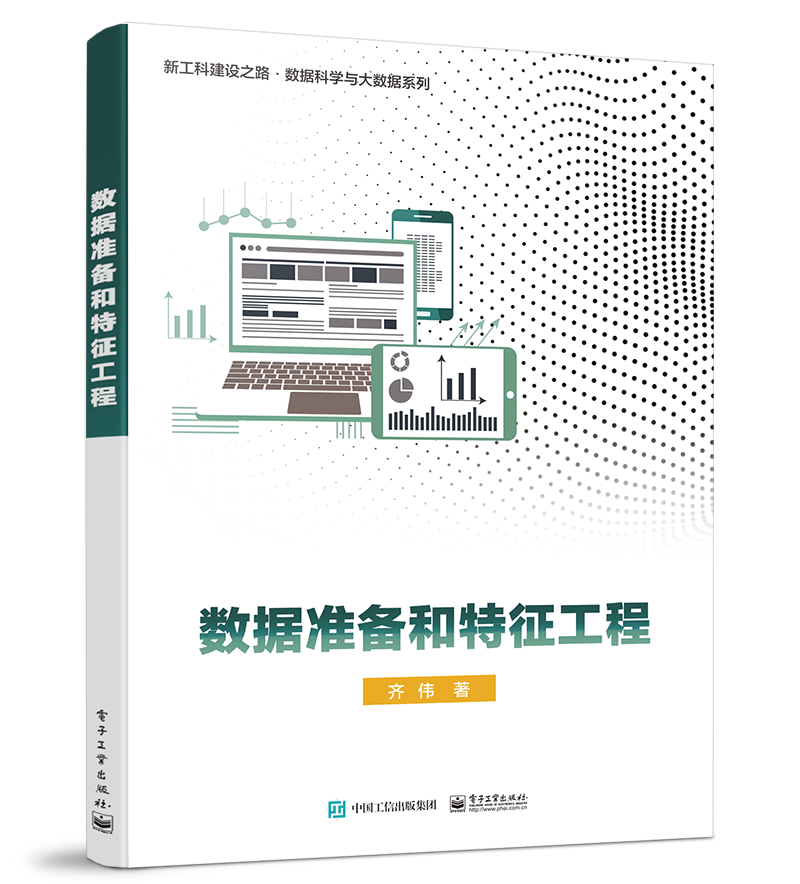## 基本概念

### KL散度

KL散度用于度量一个概率分布p偏离另一个期望的概率分布q的程度，它是不对称的。

\begin{aligned}D_{KL}(p | q) &= \sum_x p(x) \log \frac{p(x)}{q(x)} dx \ &= - \sum_x p(x)\log q(x) + \sum_x p(x)\log p(x) \ &= H(P, Q) - H(P)\end{aligned}

### 互信息

\begin{aligned} I(X;Y) &= D_{KL}[p(x,y) | p(x)p(y)] \ &= \sum_{x \in X, y \in Y} p(x, y) \log(\frac{p(x, y)}{p(x)p(y)}) \ &= \sum_{x \in X, y \in Y} p(x, y) \log(\frac{p(x|y)}{p(x)}) \ &= H(X) - H(X|Y) \ \end{aligned}

## 马尔科夫链的深度神经网络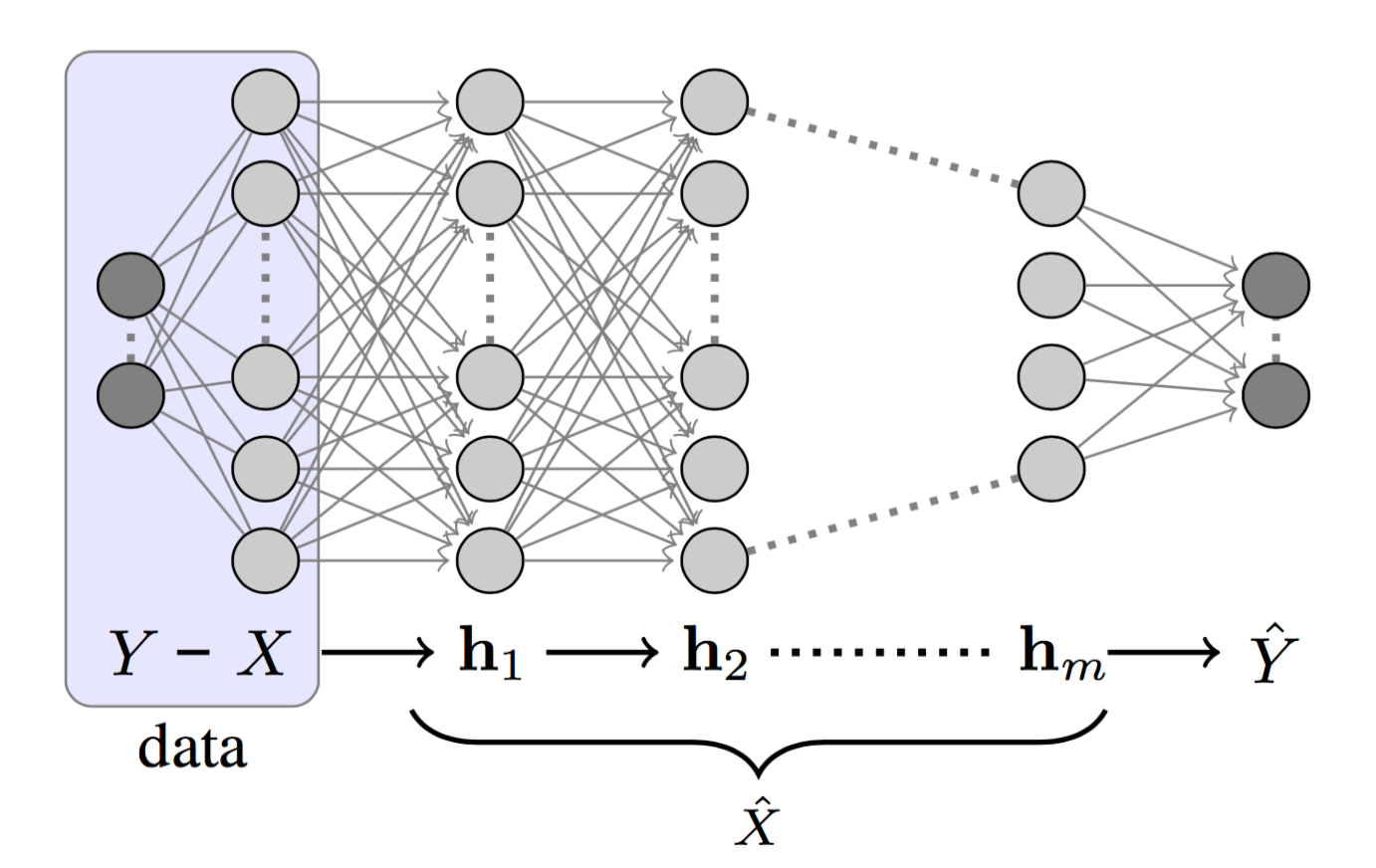$$H(X) \geq I(X; h_1) \geq I(X; h_2) \geq \dots \geq I(X; h_m) \geq I(X; \hat{Y}) \ I(X; Y) \geq I(h_1; Y) \geq I(h_2; Y) \geq \dots \geq I(h_m; Y) \geq I(\hat{Y}; Y)$$

DNN的设计目的是学习如何描述$X$，以便预测$Y$；最终，将$X$压缩成只包含与$Y$相关的信息。Tishby将这一过程描述为“相关信息的逐次细化”。

### 信息平面定理

DNN依次实现了$X$的内部表示，一组隐藏层${T_i}$。根据信息平面定理，通过它的编码器和解码器信息来描述每一层，编码器即对输入数据$X$编码，而解码器则将当前层中的信息转换为目标输出$Y$。

• X轴：样本$T_i$复杂度由编码器互信息$I(X;T_i)$决定，样本复杂度是指你需要多少个样本来达到一定的准确性和泛化。
• Y轴：精度(泛化误差)，由解码器互信息$I(T_i;Y)$决定。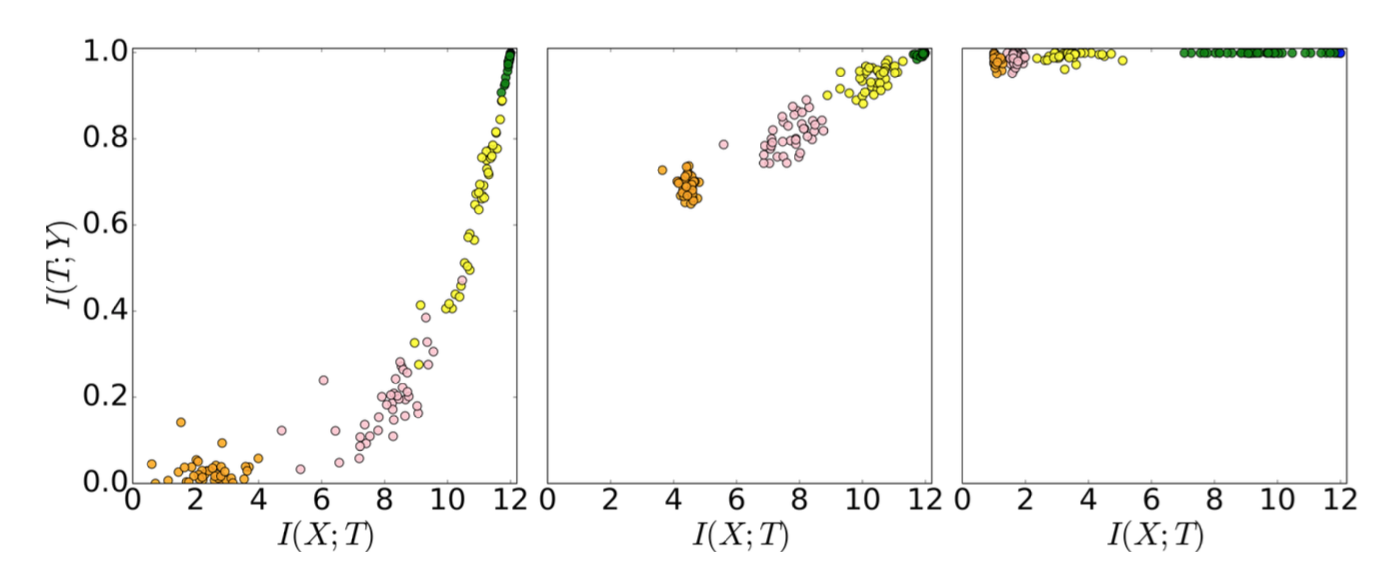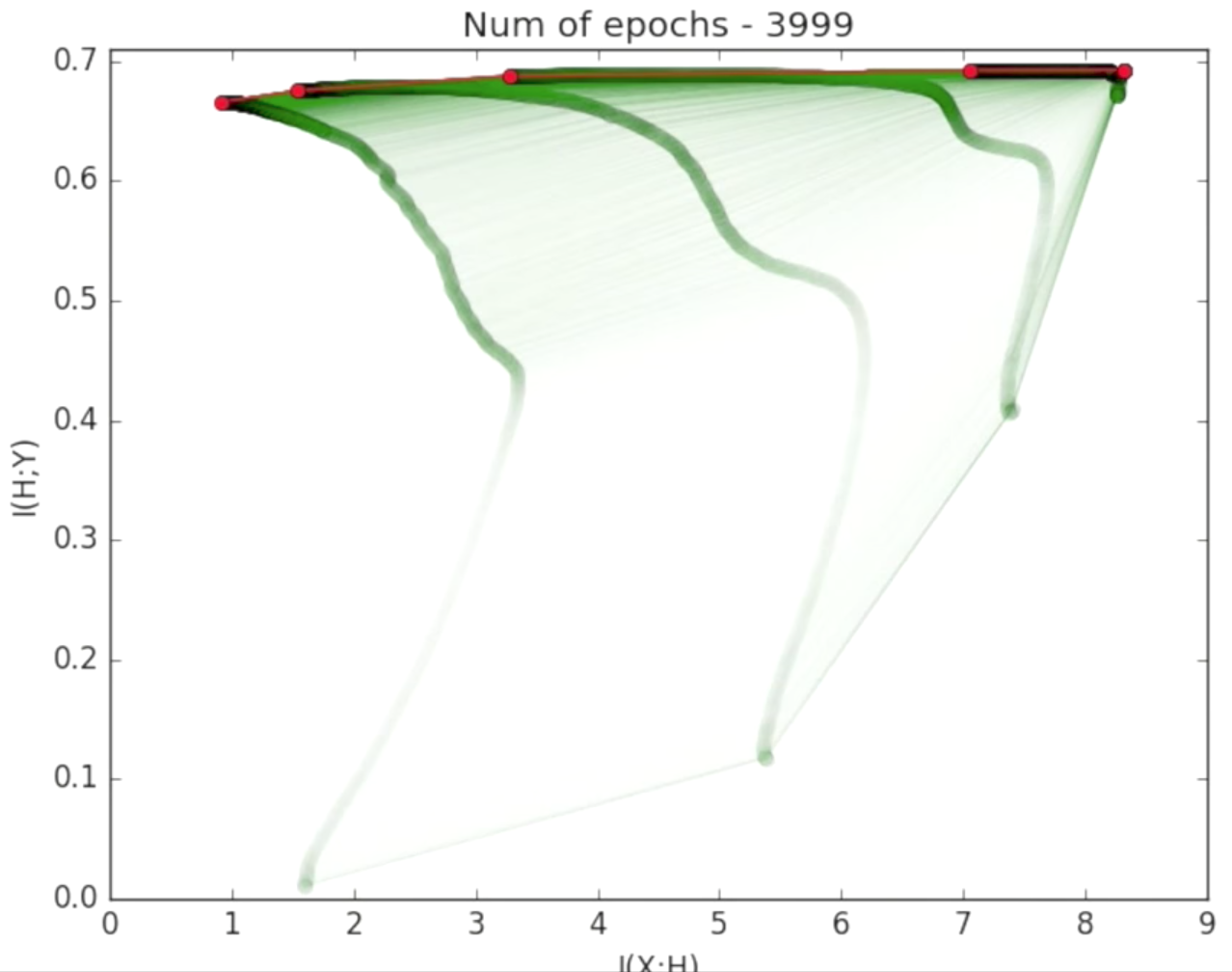### 两个优化阶段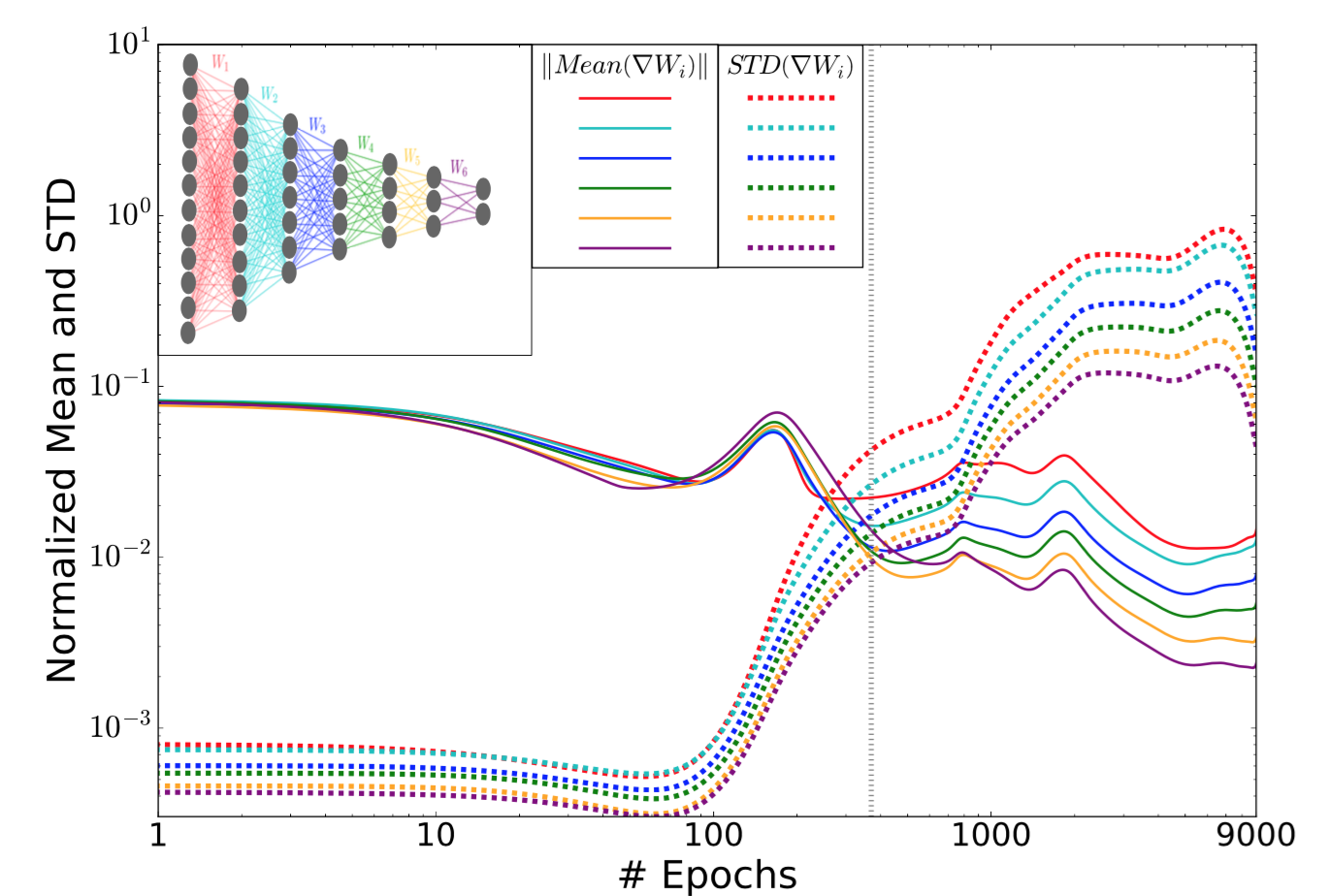## 学习理论

### “旧的”泛化

$$\epsilon^2 < \frac{\log|H_\epsilon| + \log{1/\delta}}{2m}$$

• $\epsilon$：训练误差与泛化误差的差值。泛化误差衡量的是一个算法对前所未见的数据的预测有多准确。
• $H_\epsilon$: 假设，通常我们假设大小为$\vert H_\epsilon \vert \sim (1/\epsilon)^d$.
• $\delta$: 信度。
• $m$: 训练样本的数目。
• $d$：假设的VC维度。

### “新的”输入压缩

$$|H_\epsilon| \sim 2^{|X|} \to 2^{|T_\epsilon|}$$

$$\epsilon^2 < \frac{2^{I(T_\epsilon; X)} + \log{1/\delta}}{2m}$$## 网络和训练数据的大小

### 更多隐藏层的好处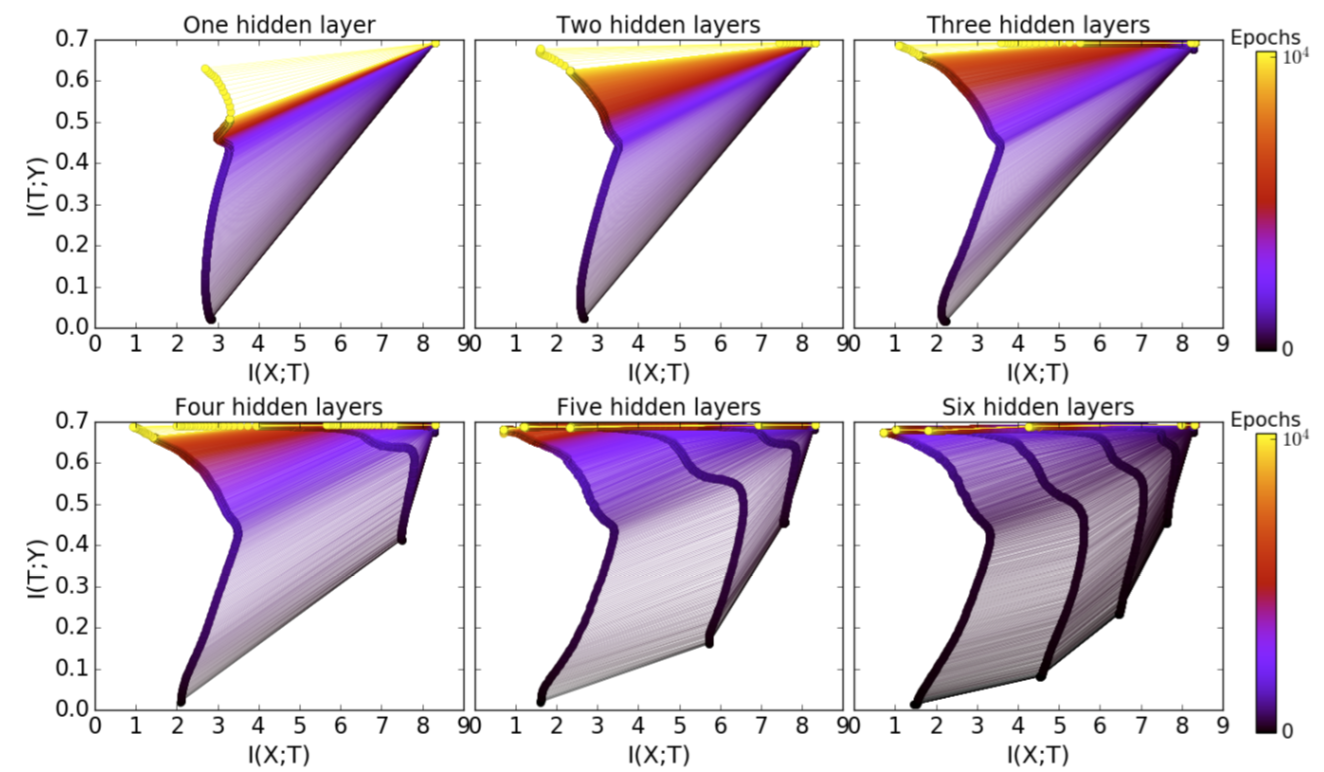### 更多训练样本的好处